In this article, we will begin our acquaintance with graphs, get acquainted with the breadth-first search algorithm (BFS) and implement the graph in the Rust programming language.

## What is a graph?

The graph reproduces a set of connections. Imagine that there is Mark, who has heard a lot about Eva and now wants to get to meet her. Mark has two friends, Alice and Andrew. Alice knows Eve through her friend Monika, and Andrew knows Eve directly. Such formulations of connections are difficult to perceive, but you can easily display this connection using a graph. Arrows show the direction of connections: Mark friends with Alice and Andrew, Alice friends with Monika, etc.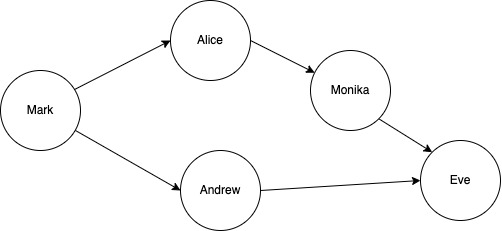Each graph consists of nodes and edges.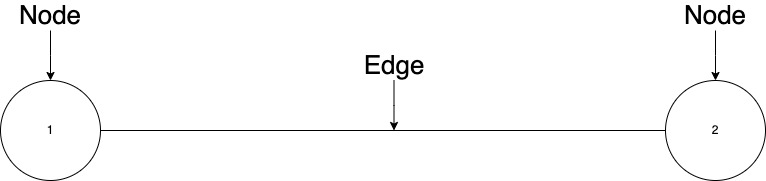## Types of graphs

There are many types of graphs, here are some of them:

• undirected;
• oriented;
• multigraph;
• pseudograph.

Let's look at their graphical representation.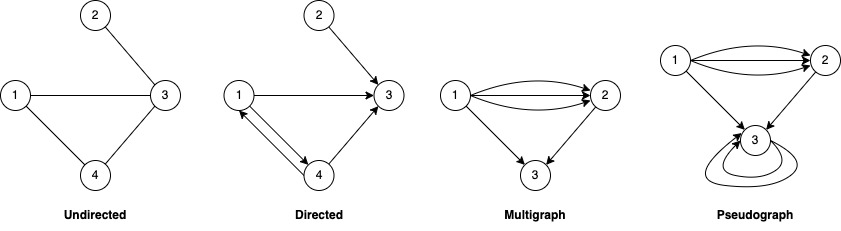## Representation of graphs in code

There are four main methods of graph representation. The choice of representation is determined by the conditions of a specific task. They differ in the amount of memory occupied and the speed of operations on graphs. There are different combinations of graph components:

• The adjacency matrix of the graph (or the matrix of weights of the graph).
• Structures (lists) of adjacent graph vertices.
• The incidence matrix of the graph: vertex–edges.
• List of edges of the graph.

The adjacency matrix and lists of adjacent vertices are most often used. However, it should be emphasized that with a small number of nodes ~ 10, the representation does not matter.

In the following examples, I will use the adjacency matrix, but in the work use the representation that is most effective for your task.

The adjacency matrix of a graph with n nodes is called the matrix Anxn, whose elements:

• If node i is adjacent to node j, then the value = 1.
• If node i is not adjacent to node j, then the value = 0.
• If there are k number of edges coming from the node, then the value = k.
• If the node has v loops, then the corresponding value on the diagonal = v, if without loops = 0.

This is how the matrix for graphs from the previous section will look like: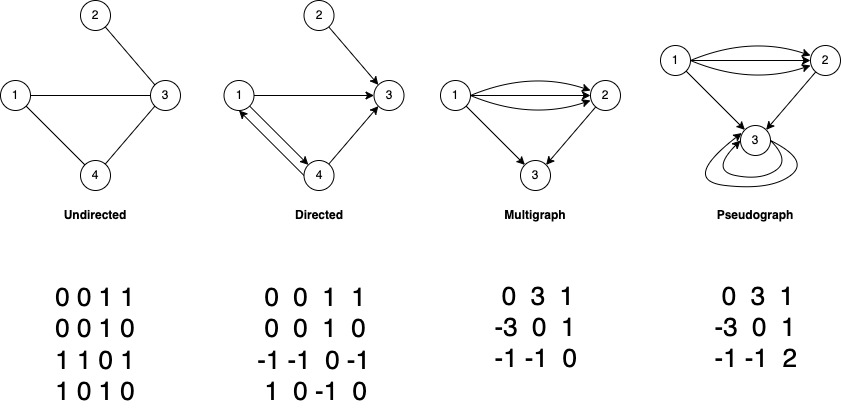In a directed graph, both 0 and -1 can be used to indicate the occurrence of a node. For example, for the second graph, in the figure above, the edge (3,1) can be denoted in the matrix either -1 or 0. In the examples I will use 0.

Let's implement the matrix in the Rust programming language.

```use std::fmt::{Display, Formatter, Result, Write};
use std::ops::{Index, IndexMut};
use std::vec;

#[derive(Clone)]
pub struct Row {
data: Vec<i32>,
}

impl Row {
pub fn new(size: usize, filler: i32) -> Self {
Self {
data: vec![filler; size],
}
}
}

impl Index<usize> for Row {
type Output = i32;
fn index(&self, index: usize) -> &Self::Output {
&self.data[index]
}
}

impl IndexMut<usize> for Row {
fn index_mut(&mut self, index: usize) -> &mut Self::Output {
&mut self.data[index]
}
}

impl Display for Row {
fn fmt(&self, f: &mut Formatter) -> Result {
let mut str_row = String::new();
self.data
.iter()
.for_each(|c| write!(&mut str_row, "{} ", c).unwrap());
write!(f, "{}", str_row.trim_end())
}
}
```

Well, now we describe the structure of the matrix and the basic functions for working with adjacent nodes.

```pub struct Matrix {
/// Number of nodespub n: usize,
data: Vec<Row>,
}

impl Index<usize> for Matrix {
type Output = Row;
fn index(&self, index: usize) -> &Self::Output {
&self.data[index]
}
}

impl IndexMut<usize> for Matrix {
fn index_mut(&mut self, index: usize) -> &mut Self::Output {
&mut self.data[index]
}
}

impl Matrix {

/// Creates a new instance of Matrix

/// with n nodes

pub fn new(nodes: usize, filler: i32) -> Self {
Self {
n: nodes,
data: vec![Row::new(nodes, filler); nodes],
}
}
}

impl Matrix {

pub fn add_weighted_edge(&mut self, n: usize, m: usize, val: i32) {﻿
self[n][m] = val;
}

/// Adds a directed edge without weight

pub fn add_directed_edge(&mut self, n: usize, m: usize) {﻿
}

/// Adds a directed edge with weight

pub fn add_w_directed_edge(&mut self, n: usize, m: usize, w: i32) {
}

pub fn add_undirected_edge(&mut self, n: usize, m: usize) {
}
}

impl Matrix {

/// Returns true if some node has adjacent nodes

pub fn has_adjs(&self, node: usize) -> bool {﻿
}

﻿pub fn get_adjs(&self, node: usize) -> Vec<usize> {
for j in 0..self.n {
if self[node][j] > 0 {
}
}
}

pub fn adjs_count(&self, node: usize) -> usize {
}
}

impl Display for Matrix {
fn fmt(&self, f: &mut Formatter) -> Result {
let mut matrix = String::new();
if !self.data.is_empty() {
for row in &self.data {
matrix.push_str(&row.to_string());
matrix.push('\n');
}
} else {
matrix = String::from("Matrix is empty!");
}
write!(f, "{}", matrix)
}
}

impl Matrix {
pub fn bfs(&self, starter_node: usize, target_node: usize) -> bool {
let mut queue = vec![];
queue.push(starter_node);
let mut searched = vec![];
while !queue.is_empty() {
let node = queue.remove(0);
if !searched.contains(&node) {
if node == target_node {
println!("The target node was found!");
println!("Searched: {:?}", searched);
return true;
} else {
searched.push(node);
}
println!("Node: {:?}", node);
println!("Q: {:?}\n", queue);
}
}
return false;
}
}
```

Now is the time to consider one of the graph traversal algorithms - "Breadth-first Search".

## BFS algorithm

This algorithm allows us to answer two questions:

1. Is there a path from one node to another?
2. Which is the shortest way?

Let's assume that our task is to find an influencer in the field of programming, among our friends and their friends who form a network of contacts. This is what our network looks like: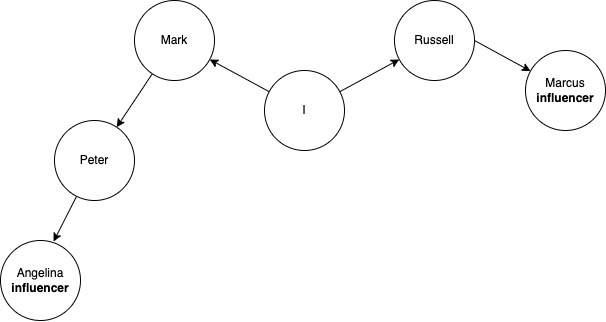There are two influencers in our network of contacts: Marcus and Angelina, but we still don't about this.

We need to answer two questions:

1. Is there an influencer in our network?
2. What is the shortest way to the influencer?

Our graph has levels, let's reflect on them.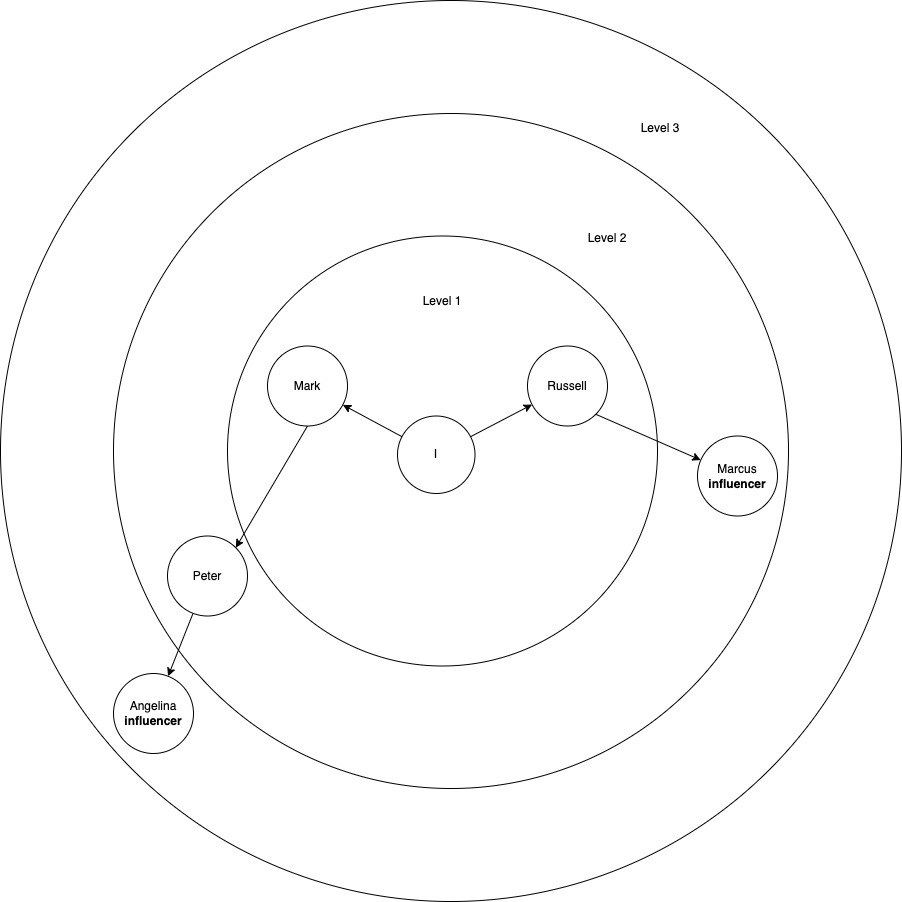The algorithm starts from level one and moves on to the next levels as needed.

Abstractly, the algorithm looks like this:

1. We ask Mark and Russell if they are influencers. If one of them is an influencer, then we stop the algorithm on the first found influencer. If there are no influencers among them, then we ask them to share the question with their friends. Mark and Russell are not influencers, we go to their friends to the next level.
2. Mark and Russell ask Peter and Marcus if they are influencers. If one of them is an influencer, then we stop the algorithm on the first found influencer. If there are no influencers among them, then we ask you to repeat the request among their friends. Marcus is an influencer, so we stop the algorithm.

Let's program the implementation. We will use recursion, since it is clear from the text above that they perform the same action.

Let's program the implementation. We will use queues.

```impl Matrix {
pub fn bfs(&self, starter_node: usize, target_node: usize) -> bool {
let mut queue = vec![];
queue.push(starter_node);
let mut searched = vec![];
while !queue.is_empty() {
let node = queue.remove(0);
if !searched.contains(&node) {
if node == target_node {
println!("The target node was found!");
println!("Searched: {:?}", searched);
return true;
} else {
searched.push(node);
}
println!("Node: {:?}", node);
println!("Q: {:?}\n", queue);
}
}
return false;
}
}
```

Well, now let's create our graph and go through it with a search in width.

```fn main() {
// Node 0 - Me

// Node 1 - Russell

// Node 2 - Mark

// Node 4 - Marcus

// Node 3 - Peter
﻿
// Node 5 - Angelina
﻿
let mut graph: Matrix = Matrix::new(6, 0);
graph.bfs(0, 4);
}
```

And this is what we get at the output:

```Node: 0
Q: [1, 2]

Node: 1
Q: [2, 4]

Node: 2
Q: [4, 3]

The target node was found!
Searched: [0, 1, 2]
```

After passing the first level, nodes 1 and 2, the first in the queue was the desired node 4, which the algorithm reported and stopped its execution.

The source code can be found in my repository: https://github.com/arg2u/graphula

## Conclusion

A graph is one of the fundamental structures for programmers. It is used for modeling different relations between objects.

Different graphics algorithms help to find a short path from A to B in the navigation maps, to find the most effective solution in complex systems and others.

Hashtags:
#graphs
#algorithms
#rust
Share:

## Lates

### Composition of the IT development team

In this article we will look at the composition of the IT solution development team

#developmentprocess

### What is the difference between outsourcing development and outstaffing an IT employee for development?

In this article we will understand what outsourcing and outstaff development are.

#developmentprocess

### UI/UX design: The creation process

In this article we will talk about the main steps in the process of creating UI/UX design.

#developmentprocess

### UI/UX design: Introduction

In this article, we begin to get acquainted with UI / UX design. This is the most important stage in the development of any visual application interface.

#developmentprocess

### Agile, Six Sigma and No Principle

In the last article, we started diving into the development process. The first stage of this process is planning. At this stage, the project manager, together with other team members, forms a pool of tasks in accordance with some kind of project management methodology.

#developmentprocess

### Meet the Pentest

We are beginning to consider one of the main methods of assessing the security of computer systems and networks for potential vulnerabilities - penetration testing

#pentest

### Reducing the implementation period of MVP

Let's figure out the timing of the implementation of the MVP.

#developmentprocess

#wannacode

### Testing an MVP concept

We will figure out how not to waste the budget on MVP development in vain

#developmentprocess

### Application Architecture Design: Introduction

In this article, we will talk about the process of creating the architecture of an IT solution.

#developmentprocess

### The terms of references: Structure

In this publication we will consider the universal structure of ToR

#developmentprocess

### Incorrect estimation of the cost of IT contractor services

Today we will talk about the incorrect assessment of the cost of developing IT solutions. This pain is one of the main ones for enterprises and startups, including IT contractors themselves.

#consultin

### Introduction to Design Patterns in Software Development

In this article, we will begin to dive into the world of optimizing application architecture using design patterns.

#designpatterns

### Choosing the direction of development for programming training

In this article, you will find out what areas of IT development there are, how they differ and in which they pay more

#wannacode

### OSI Model Levels

In this article, we will take a closer look at each of the levels of the OSI model

### Main types of application architecture

In this publication, we will look at what application architectures are

#developmentprocess

### 10 ways to use Rust Cargo

In this short article I have collected 10 ways to use the build system and package manager of the Rust programming language

### Documenting code in the Rust programming language

In this article, we will look at how documentation takes place in Rust and consider a very useful opportunity - writing tests through documentation.

#rust

### Introduction to the OSI model

In this article we begin to consider the fundamental model of network interaction - OSI

#networks

### CSS animation ripple

A simple example of how to implement ripple animation using HTML and CSS

#css

### What is the purpose of an ER-diagram in the development process?

Let's discuss in general terms what an ER diagram is and what it is used for.

#developmentprocess

### From concept to MVP

In this article, you will learn, by example, how to move from a concept to an MVP without unnecessary complications in the functionality of the product

#developmentprocess

### What are UML diagrams used for?

In this article we will talk about what UML diagrams are, what they are and where they are used

#developmentprocess

### Introduction to writing the terms of references

The Terms of Reference are an important part of the development process. In this article, we will begin to dive into this issue.

#developmentprocess

### Introduction to software development

Today, most companies are faced with IT development and often do not get what they want. In this article, we begin to dive into the process of creating IT solutions.

#developmentprocess

### From idea to concept

In this publication, we will talk about how the idea differs from the concept. Let's do this with an example of a specific goal

#developmentprocess

### IT project management methodologies: Waterfall, Scrum, Prince2

In this article, we will consider the basic methodologies of IT project management.

#developmentprocess

### Weighted graphs

In this article, we will get acquainted with weighted graphs, Dijkstra's algorithm, and its implementation in the Rust programming language.

### Development Process: Planning

In this publication, we will begin to consider the development process. Let's start with the planning process.

#developmentprocess